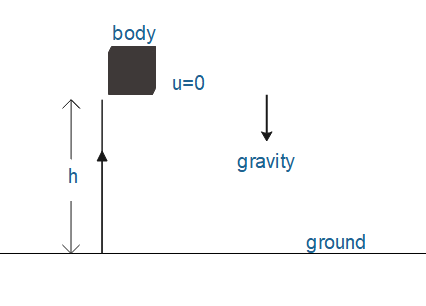QuestionAnswers

# A body falls freely under gravity from rest and reaches the ground in time t. Write an expression for the height fallen by the body.\begin{align} & \text{A}\text{. H=}\dfrac{1}{2}g{{t}^{2}} \\ & \text{B}\text{. H=ut+}\dfrac{1}{2}g{{t}^{2}} \\ & \text{C}\text{. H=}\dfrac{1}{2}gt \\ & \text{D}\text{. H=ut-}\dfrac{1}{2}g{{t}^{2}} \\ \end{align}Verified
128.7k+ views
Hint: Use a second equation of motion which gives relation between$g,H,u\text{ and }t$. Acceleration due to gravity plays a role over here because the body is under the influence of gravity. At starting position, the body is at rest therefore initial velocity must be zero.

Formula used:
We know that second equation of motion is given by
$H=ut+\dfrac{1}{2}a{{t}^{2}}$
Where,
H=distance
u=initial velocity
t=time
g=acceleration due to gravityWe know that second equation of motion is given by
$H=ut+\dfrac{1}{2}a{{t}^{2}}$
Where, H=distance, u=initial velocity, t=time, g=acceleration due to gravity
Since the body is at rest therefore value of initial position must be zero.
$\text{i}\text{.e}\text{. }u=0$
$H=\dfrac{1}{2}g{{t}^{2}}$
The height fallen by the body is $H=\dfrac{1}{2}g{{t}^{2}}$.

So the correct answer is Option A.

Air resistance is the one of the types of force which air exerts on objects moving in the surrounding. It is often referred to as drag force. In free fall cases, generally air resistance is neglected. Mainly, air resistive force directed opposite to the object's motion, therefore sowing it down. Whenever we consider free fall cases, we usually neglect resistance to air.
Suppose the acceleration of a particle is $a$ and remains constant. Let the velocity at time 0 be $u$ and the velocity at time $t$ be $v$. Thus,
\begin{align} & \dfrac{dv}{dt}=a\text{ or }dv=adt \\ & \text{or }\int\limits_{u}^{v}{dv=\int\limits_{0}^{t}{adt}} \\ \end{align}
As time changes from 0 to $t$the velocity changes from $u$ to $v$. So on the left hand side the summation is made over $v$ from $u$ to $v$ whereas on the right hand side the summation is made on time from 0 to $t$. Evaluating the integrals we get,
\begin{align} & \left[ v \right]_{u}^{v}=a\left[ t \right]_{0}^{t} \\ & v-u=at \\ & v=u+at----(1) \\ \end{align}
Above equation can be written as
\begin{align} & \dfrac{dH}{dy}=u+at \\ & dH=(u+at)dt \\ \end{align}
Now intergrate dH and dt from 0 to H and 0 to t respectively

$\int\limits_{0}^{H}{dH}=\int\limits_{0}^{t}{(u+at)dt}$

At $t=0$ the particle is at $\text{H }=\text{ }0$. As time changes from 0 to $t$ the position changes from 0 to $H$. So on the left hand side the summation is made on position from 0 to $H$ whereas on the right hand side the summation is made on time from 0 to $t$. Evaluating the integrals, the above equation becomes
\begin{align} & \left[ H \right]_{0}^{H}=\int\limits_{0}^{t}{udt}+\int\limits_{0}^{t}{utdt} \\ & H=ut+\dfrac{1}{2}a{{t}^{2}}--(2) \\ \end{align}
From equation one, we get
\begin{align} & {{v}^{2}}={{(u+at)}^{2}} \\ & {{v}^{2}}={{u}^{2}}+2uat+{{a}^{2}}{{t}^{2}} \\ & {{v}^{2}}={{u}^{2}}+2aH---(3) \\ \end{align}
From equation (1), (2) and (3)
\begin{align} & v=u+at \\ & H=ut+\dfrac{1}{2}a{{t}^{2}} \\ & {{v}^{2}}={{u}^{2}}+2aH \\ \end{align}

Note:
The quantities $u,v\text{ and a}$ may take positive or negative values on whether they are directed along the positive or negative direction. Similarly H may be positive or negative. Remember that $H$ represents the position of the particle at time $t$ and not (in general) the distance travelled by it in time 0 to $t$.